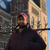QlikView App Dev

Discussion Board for collaboration related to QlikView App Development.

Announcements
QlikWorld 2022, LIVE in Denver CO., May 16-19, 2022. REGISTER NOW TO RECEIVE EARLY BIRD PRICING
cancel
Showing results for
Did you mean:Creator II

If Logic in script

Hi All,

I am trying to move 1 logic from front end to back end.

Front end Logic -

Count(Distinct{

<T_Time_ID ={">=7"},Policy_Type_Flag={2},Status_Flag={2},PaymentGroup={2,3},OutstandingBalance_Flag={1}>

+

<T_Time_ID ={">=7"},Policy_Type_Flag={2},Status_Flag={2},PaymentGroup={1,4}>

} P_Q_ID)

Back end -

If(T_Time_ID >=7,

If(Policy_Type_Flag=2,

If(Status_Flag=2,If(PaymentGroup=2 OR PaymentGroup=3,IF(OutstandingBalance_Flag= 1,1,0),0),0),0),0),

IF(T_Time_ID >=7,

If(Policy_Type_Flag=2,

If(Status_Flag=2,If(PaymentGroup=1 OR PaymentGroup=4,1,0),0),0),0) as CurrentFlag

I am not sure how to put the condition in backend as 1 condition required outstanding flag and 1 not.

Thanks

Neha

1 Solution

Accepted SolutionsAnonymous
Not applicable

try this:

If((T_Time_ID >=7) and (Policy_Type_Flag=2) and (Status_Flag=2),

If (((PaymentGroup=2) OR (PaymentGroup=3)) and (OutstandingBalance_Flag= 1),1,

If ((PaymentGroup=1) OR (PaymentGroup=4),1 ,0))) as CurrentFlag

3 RepliesChampion

Hi,

I would've tried this:

if( (T_Time_ID >= 7 and Policy_Type_Flag = 2 and Status_Flag = 2 and Match(PaymentGroup,2,3) and OutstandingBalance_Flag = 1) OR (T_Time_ID >=7 and Policy_Type_Flag = 2 and Status_Flag = 2 and Match(PaymentGroup,1,4) ), 1, 0) as CurrentFlagAnonymous
Not applicable

try this:

If((T_Time_ID >=7) and (Policy_Type_Flag=2) and (Status_Flag=2),

If (((PaymentGroup=2) OR (PaymentGroup=3)) and (OutstandingBalance_Flag= 1),1,

If ((PaymentGroup=1) OR (PaymentGroup=4),1 ,0))) as CurrentFlagCreator II
Author

Hi,

This works fine, Thanks.

NehaCommunity Browser